# ISEE Lower Level Quantitative : How to compare fractions

## Example Questions

### Example Question #2741 : Isee Lower Level (Grades 5 6) Quantitative Reasoning

Select the symbol to correctly fill in the blank below.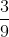__________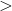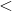Explanation:

To compare fractions, we need to first make common denominators.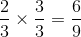Now that we have common denominators, we can compare numerators. The fraction with the bigger numerator has the greater value.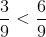### Example Question #2742 : Isee Lower Level (Grades 5 6) Quantitative Reasoning

Select the symbol to correctly fill in the blank below.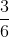__________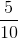Explanation:

To compare fractions, we need to first make common denominators.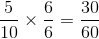Now that we have common denominators, we can compare numerators. The fraction with the bigger numerator has the greater value.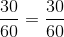### Example Question #2743 : Isee Lower Level (Grades 5 6) Quantitative Reasoning

Select the symbol to correctly fill in the blank below.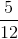__________Explanation:

To compare fractions, we need to first make common denominators.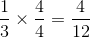Now that we have common denominators, we can compare numerators. The fraction with the bigger numerator has the greater value.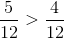### Example Question #2744 : Isee Lower Level (Grades 5 6) Quantitative Reasoning

Select the symbol to correctly fill in the blank below.__________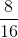Explanation:

To compare fractions, we need to first make common denominators.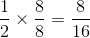Now that we have common denominators, we can compare numerators. The fraction with the bigger numerator has the greater value.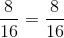### Example Question #2745 : Isee Lower Level (Grades 5 6) Quantitative Reasoning

Select the symbol to correctly fill in the blank below.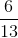__________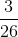Explanation:

To compare fractions, we need to first make common denominators.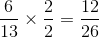Now that we have common denominators, we can compare numerators. The fraction with the bigger numerator has the greater value.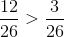### Example Question #2746 : Isee Lower Level (Grades 5 6) Quantitative Reasoning

Select the symbol to correctly fill in the blank below.__________Explanation:

To compare fractions, we need to first make common denominators.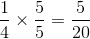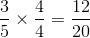Now that we have common denominators, we can compare numerators. The fraction with the bigger numerator has the greater value.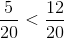### Example Question #41 : Number & Operations: Fractions

Select the symbol to correctly fill in the blank below.__________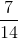Explanation:

To compare fractions, we need to first make common denominators.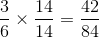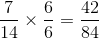Now that we have common denominators, we can compare numerators. The fraction with the bigger numerator has the greater value.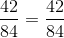### Example Question #42 : Number & Operations: Fractions

Select the symbol to correctly fill in the blank below.__________Explanation:

To compare fractions, we need to first make common denominators.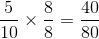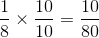Now that we have common denominators, we can compare numerators. The fraction with the bigger numerator has the greater value.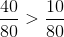### Example Question #43 : Number & Operations: Fractions

Select the symbol to correctly fill in the blank below.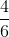__________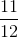Explanation:

To compare fractions, we need to first make common denominators.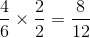Now that we have common denominators, we can compare numerators. The fraction with the bigger numerator has the greater value.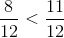### Example Question #44 : Number & Operations: Fractions

Select the symbol to correctly fill in the blank below.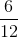__________Explanation:

To compare fractions, we need to first make common denominators.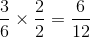Now that we have common denominators, we can compare numerators. The fraction with the bigger numerator has the greater value.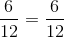### All ISEE Lower Level Quantitative Resources